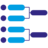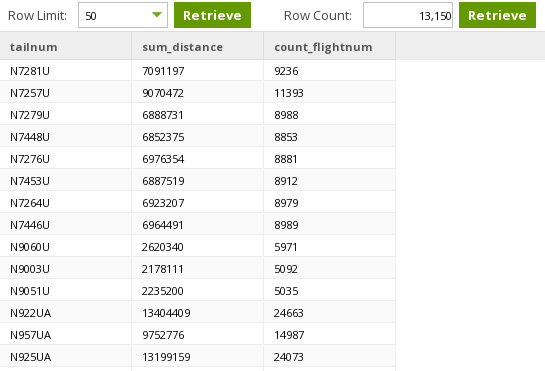• Matillion ETL v1.64
• Matillion ETL
• Hub
Aggregate
• Dark
Light

# Aggregate

• Dark
Light## Aggregate Component

The Aggregate component groups together multiple input rows into a single output row. Input columns can be added to the groupings, or have an aggregation applied to them.

Default output names are chosen by combining the source column name and the aggregation type. If they are not appropriate, consider using a Rename Component immediately after the Aggregate component to choose more appropriate names.

## Snowflake Properties

Property Setting Description
Name String Specify A human-readable name for the component.
Groupings Column Select Select one or more columns from the source table that will form the groupings.
The output flow will contain one row for each distinct combination of values within the grouping columns.
Aggregations Source Columns Select the input (source) column for the summary function (the summary function is the aggregation type).
Aggregation Type Users can add the same source column multiple times, each with a different aggregation type. The summary is calculated for each combination of the source column values.
Min: Find the Minimum value of the source column.
Max: Find the Maximum value of the source column.
Count: Count the total number of records whose source column is not null.
Count Distinct: Count the total number of distinct (unique) values of the source column.
Approximate Count: This is a variant of Count Distinct that may have better performance in cases where an exact count is not required. Sum: Sum the values in the source column. This is only sensible for numeric source columns.
Average: Average the values in the source column. This is only sensible for numeric source columns.
Standard Deviation (sample): Calculates the sample standard deviation of the source column. This is only sensible for numeric source columns.
Standard Deviation (population): Calculates the population standard deviation of the source column. This is only sensible for numeric source columns.
Variance (sample): Calculates the sample variance of the source column. This is only sensible for numeric source columns.
Variance (population): Calculates the population variance of the source column. This is only sensible for numeric source columns.
Median: Calculates the median value for the range of values. For more information, refer to the Snowflake Median documentation.
Grouping Type Select Select how to group rows.
Group By: Groups rows with the same group-by-item expressions and computes aggregate functions for the resulting group. A GROUP BY expression can be a column name, a number referencing a position in the SELECT list, or a general expression.
Group By Cube: GROUP BY CUBE is an extension of the GROUP BY clause similar to GROUP BY ROLLUP. In addition to producing all the rows of a GROUP BY ROLLUP, GROUP BY CUBE adds all the "cross-tabulations" rows. Sub-total rows are rows that further aggregate whose values are derived by computing the same aggregate functions that were used to produce the grouped rows.
Group By Grouping Sets: GROUP BY GROUPING SETS is a powerful extension of the GROUP BY clause that allows computing multiple group-by clauses in a single statement. The group set is a set of dimension columns.
Group By Rollup: GROUP BY ROLLUP is an extension of the GROUP BY clause that produces sub-total rows (in addition to the grouped rows). Sub-total rows are rows that further aggregate whose values are derived by computing the same aggregate functions that were used to produce the grouped rows.

## Redshift Properties

Property Setting Description
Name String Specify A human-readable name for the component.
Groupings Column Select Select one or more columns from the source table that will form the groupings.
The output flow will contain one row for each distinct combination of values within the grouping columns.
Aggregations Source Columns Select the input (source) column for the summary function (the summary function is the aggregation type).
Aggregation Type Users can add the same source column multiple times, each with a different aggregation type. The summary is calculated for each combination of the source column values.
Min: Find the Minimum value of the source column.
Max: Find the Maximum value of the source column.
Count: Count the total number of records whose source column is not null.
Count Distinct: Count the total number of distinct (unique) values of the source column.
Approximate Count: This is a variant of Count Distinct that may have better performance in cases where an exact count is not required. See the Redshift documentation for more details.
Sum: Sum the values in the source column. This is only sensible for numeric source columns.
Average: Average the values in the source column. This is only sensible for numeric source columns.
Standard Deviation (sample): Calculates the sample standard deviation of the source column. This is only sensible for numeric source columns.
Standard Deviation (population): Calculates the population standard deviation of the source column. This is only sensible for numeric source columns.
Variance (sample): Calculates the sample variance of the source column. This is only sensible for numeric source columns.
Variance (population): Calculates the population variance of the source column. This is only sensible for numeric source columns.
Median: Calculates the median value for the range of values. For more information, refer to theRedshift Median documentation.
For more information on the Aggregation Types, refer the Redshift Aggregate Functions documentation.

## BigQuery Properties

Property Setting Description
Name String Specify A human-readable name for the component.
Groupings Column Select Select one or more columns from the source table that will form the groupings.
The output flow will contain one row for each distinct combination of values within the grouping columns.
Aggregations Source Columns Select the input (source) column for the summary function (the summary function is the aggregation type).
Aggregation Type Users can add the same source column multiple times, each with a different aggregation type. The summary is calculated for each combination of the source column values.
Min: Find the Minimum value of the source column.
Max: Find the Maximum value of the source column.
Count: Count the total number of records whose source column is not null.
Count Distinct: Count the total number of distinct (unique) values of the source column.
Approximate Count: This is a variant of Count Distinct that may have better performance in cases where an exact count is not required.
Sum: Sum the values in the source column. This is only sensible for numeric source columns.
Average: Average the values in the source column. This is only sensible for numeric source columns.
Standard Deviation (sample): Calculates the sample standard deviation of the source column. This is only sensible for numeric source columns.
Standard Deviation (population): Calculates the population standard deviation of the source column. This is only sensible for numeric source columns.
Variance (sample): Calculates the sample variance of the source column. This is only sensible for numeric source columns.
Variance (population): Calculates the population variance of the source column. This is only sensible for numeric source columns.
For more information on Aggregation functions in BigQuery, see the BigQuery documentation.
Array Aggregate: Constructs an array from column data. See the BigQuery documentation for more information.

## Synapse Properties

Property Setting Description
Name String Specify A human-readable name for the component.
Groupings Column Select Select one or more columns from the source table that will form the groupings.
The output flow will contain one row for each distinct combination of values within the grouping columns.
Aggregations Source Column Select the input (source) column for the summary function (the summary function is the aggregation type).
Aggregation Type Users can add the same source column multiple times, each with a different aggregation type. The summary is calculated for each combination of the source column values.
Available functions include:
• Approximate Count
• Average
• Count
• Count Distinct
• Max
• Min
• Standard Deviation - Sample
• Standard Deviation - Population
• Sum
• Variance - Sample
• Variance - Population
• Average Distinct
• Standard Deviation Distinct
• Standard Deviation Population Distinct
• Sum Distinct
• Variance Distinct
• Variance Population Distinct

## Delta Lake Properties

Property Setting Description
Name String A human-readable name for the component.
Groupings Column Select The column to be used in the Group By clause. The output flow will contain one row for each distinct combination of values within the grouping columns.
Aggregations Source Columns Select the input (source) column for the summary function (the summary function is the aggregation type).
Aggregation Type Users can add the same source column multiple times, each with a different aggregation type. The summary is calculated for each combination of the source column values.
Available functions include:
• Approximate Count
• Average
• Count
• Count Distinct
• Max
• Min
• Standard Deviation - Sample
• Standard Deviation - Population
• Sum
• Variance - Sample
• Variance - Population
• Sum Distinct
• Skewness
• Kurtosis

## Strategy

The Aggregate component generates summary (aggregate) functions along with a group-by clause.

## Example

This job counts the number of flights and totals the distance travelled for each tail number in the input data.Groupings are set to Tail Number (tailnum).Aggregations are set to total the distance, and count the records whose flight number is not null.The sample data confirms that the data is being summarised as expected.